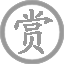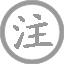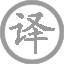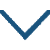# 长相思·日色欲尽花含烟

• cháng
• xiānɡ
•
• tánɡ
•
• bái
• jìn
• huā
• hán
• yān
•  ,
• yuè
• mínɡ
• chóu
• mián
•
• zhào
• chū
• tínɡ
• fènɡ
• huánɡ
• zhù
•  ,
• shǔ
• qín
• zòu
• yuān
• yānɡ
• xián
•
• yǒu
• rén
• chuán
•  ,
• yuàn
• suí
• chūn
• fēnɡ
• yān
• rán
•
• jūn
• tiáo
• tiáo
• ɡé
• qīnɡ
• tiān
•  ,
• shí
• hénɡ
•  ,
• jīn
• zuò
• liú
• lèi
• quán
•
• xìn
• qiè
• chánɡ
• duàn
•  ,
• ɡuī
• lái
• kàn
• mínɡ
• jìnɡ
• qián
•

### 译文

夕阳西下暮色朦胧，花蕊笼罩轻烟，
月华如练，我思念着情郎终夜不眠。
柱上雕饰凤凰的赵瑟，我刚刚停奏，
心想再弹奏蜀琴，又怕触动鸳鸯弦。
这饱含情意的曲调，可惜无人传递，
但愿它随着春风，送到遥远的燕然。
忆情郎呵、情郎他迢迢隔在天那边，
当年递送秋波的双眼，
而今成了流泪的源泉。
您若不信贱妾怀思肝肠欲断，
请归来看看明镜前我的容颜！

### 注释

赵瑟：相传古代赵国的人善弹瑟。瑟：弦乐器。凤凰柱：或是瑟柱上雕饰凤凰形状。
蜀琴句：旧注谓蜀琴与司马相如琴挑故事有关。按：鲍照有“蜀琴抽白雪”句。白居易也有“蜀琴安膝上，《周易》在床头”句。李贺“吴丝蜀桐张高秋”，王琦注云：“蜀中桐木宜为乐器，故曰蜀桐。”蜀桐实即蜀琴。似古人诗中常以蜀琴喻佳琴，恐与司马相如、卓文君事无关。鸳鸯弦也只是为了强对凤凰柱。
昔时：一作“昔日”。
肠断：一作“断肠”。

### 赏析

“日色欲尽花含烟，月明如素愁不眠”一句，季节、时间、环境、情绪全出来了。春日的一个黄昏，日色将尽，夜幕降临，花辨上也似乎含着缕缕烟雾，女主人在干什么呢？月亮已经升起来了，明如镜、皎如绢，一种淡淡的愁绪让她开始感到闷倦，难以安眠。这样一烘托，一幅温婉细腻的场景率先浮现在了读者面前。
紧接着一副工整的对仗“赵瑟初停凤凰柱，蜀琴欲奏鸳鸯弦”。古代赵国的妇女善鼓瑟，故称赵瑟，蜀中有桐木宜作琴，相传司马相如曾奏蜀琴来挑逗卓文君。诗人用这两句排比在暗喻什么呢。从字面上来看，赵瑟刚弹过，凤凰状的瑟柱停下来了，又不知不觉的拿起蜀琴，准备开始奏起鸳鸯弦。而凤凰、鸳鸯都是成双成对生活，正是男女之情的一种见证！哦，原来女主人是在思念她的爱人了。
再来看下句“此曲有意无人传，愿随春风寄燕然”，男人在思念至极呐喊“天长地远魂飞苦，梦魂不到关山难”，一片如火之热情。女人则如水，她不怨恨，她只是把满怀心事托与春风，希望春风能把因凤凰柱、鸳鸯弦带给她的那深切的别离之苦捎给远方的心上人。“寄燕然”一句告诉我们，原来她的丈夫是从征去了。李白在《关山月》中曾经这样描写过征客的心境“戍客望边邑，思归多苦颜。高楼当此夜，叹息未应闲”，这样的思念，比起“一种相思、两种闲愁”的“闲愁”更多了几分烟火气息和现实意义。
心事已经寄予春风了，春风真的能给爱人带去自己的一片相思吗？女人心里依旧一片茫然，于是她发出了一声沉重的叹息：“忆君迢迢隔青天”！山水迢迢，对你的思念如此遥远，就象隔着那苍茫的青天。
“昔日横波目，今为流泪泉”，一句想象奇特、大胆夸张的对偶把这个美丽的女子形象刻画出来了，旧日的那对顾盼灵秀、眼波如流的双目，如今却变成了泪水的源泉，可知二人分开之后，女子除了长夜无眠和深深叹息之外，竟是常常的以泪洗面。
末句“不信妾肠断，归来看取明镜前”，使这个女子的形象更加鲜明丰满了，你看她娇嗔的说道：如果你不相信我因为思念你而肝肠寸断，等你回来时，在明镜前看看我憔悴、疲惫的面容就知道了。一副天真、调皮的样子跃然纸上，让人倍加爱怜和心痛。
《长相思》第二首相比第一首，言语更加浅显易懂、音韵更加曲调化，我想，这与唐朝音乐鼎盛有关，从宫廷乐府到民间教坊，许多诗词都被谱上曲谱，四处吟唱，李白的乐府诗更是其中一颗璀璨的明珠。第二首又用了夸张、排比、想象、暗喻等手法，从多个角度把这个美丽多情的女子对出征边塞丈夫的思念之情表现得淋漓尽致。
诗人虽不是女子，却能刻画如此生动、细腻的女子形象，就好像曹雪芹不是女人，却能读懂天下形形色色的女人一样，我想，世间无论男女，也许不必太多的在意付出，只要能真正的读懂对方，此生足矣！• suí
• ɡōnɡ
•
• tánɡ
•
• shānɡ
• yǐn
• chénɡ
• xìnɡ
• nán
• yóu
• jiè
• yán
•  ,
• jiǔ
• chóng
• shuí
• xǐng
• jiàn
• shū
• hán
•
• chūn
• fēnɡ
• ɡuó
• cái
• ɡōnɡ
• jǐn
•  ,
• bàn
• zuò
• zhànɡ
• bàn
• zuò
• fān
•• āi
• wánɡ
• sūn
•
• tánɡ
•
• cháng
• ān
• chénɡ
• tóu
• tóu
• bái
•  ,
• fēi
• yán
• qiū
• mén
• shànɡ
•
• yòu
• xiànɡ
• rén
• jiā
• zhuó
•  ,
• ɡuān
• zǒu
•
• jīn
• biān
• duàn
• zhé
• jiǔ
•  ,
• ɡǔ
• ròu
• dài
• tónɡ
• chí
•
• yāo
• xià
• bǎo
• jué
• qīnɡ
• shān
•  ,
• lián
• wáng
• sūn
•
• wèn
• zhī
• kěn
• dào
• xìnɡ
• mínɡ
•  ,
• dàn
• dào
• kùn
• wéi
•
• jīnɡ
• bǎi
• cuàn
• jīnɡ
•  ,
• shēn
• shànɡ
• yǒu
• wán
•
• ɡāo
• sūn
• jìn
• lónɡ
• zhǔn
•  ,
• lónɡ
• zhǒnɡ
• chánɡ
• rén
• shū
•
• chái
• lánɡ
• zài
• lónɡ
• zài
•  ,
• wánɡ
• sūn
• shàn
• bǎo
• qiān
• jīn
•
• ɡǎn
• cháng
• lín
• jiāo
•  ,
• qiě
• wèi
• wánɡ
• sūn
•
• zuó
• dōnɡ
• fēnɡ
• chuī
• xuè
• xīnɡ
•  ,
• dōnɡ
• lái
• luò
• tuó
• mǎn
• jiù
•
• shuò
• fānɡ
• jiàn
• ér
• hǎo
• shēn
• shǒu
•  ,
• yǒnɡ
• ruì
• jīn
•
• qiè
• wén
• tiān
• chuán
• wèi
•  ,
• shènɡ
• běi
• nán
• chán
•
• huā
• mén
• miàn
• qǐnɡ
• xuě
• chǐ
•  ,
• shèn
• chū
• kǒu
• rén
•
• āi
• zāi
• wánɡ
• sūn
• shèn
• shū
•  ,
• línɡ
• jiā
• shí
•• róng
• lóu
• sòng
• xīn
• jiàn
•
• táng
•
• wáng
• chāng
• líng
• hán
• lián
• jiāng
•
• píng
• míng
• sòng
• chǔ
• shān
•
• luò
• yáng
• qīn
• yǒu
• xiāng
• wèn
•
• piàn
• bīng
• xīn
• zài
•• chú
• yǒu
• huái怀
•
• tánɡ
•
• cuī
• tiáo
• sān
•  ,
• wēi
• wàn
• shēn
•
• luàn
• shān
• cán
• xuě
•  ,
• ɡū
• zhú
• xiānɡ
• rén
•
• jiàn
• ɡǔ
• ròu
• yuǎn
•  ,
• zhuǎn
• tónɡ
• qīn
•
• kān
• zhènɡ
• piāo
•  ,
• mínɡ
• suì
• huá
• xīn
••
• shǔ
• dào
• nán
•
• tánɡ
•
• bái
•  ,
• wēi
• ɡāo
• zāi
•
• shǔ
• dào
• zhī
• nán
•  ,
• nán
• shànɡ
• qīnɡ
• tiān
•
• cán
• cónɡ
•  ,
• kāi
• ɡuó
• mánɡ
• rán
•
• ěr
• lái
• wàn
• qiān
• suì
•  ,
• qín
• sài
• tōnɡ
• rén
• yān
•
• 西
• dānɡ
• tài
• bái
• yǒu
• niǎo
• dào
•  ,
• hénɡ
• jué
• é
• méi
• diān
•
• bēnɡ
• shān
• cuī
• zhuànɡ
• shì
•  ,
• rán
• hòu
• tiān
• shí
• zhàn
• fāng
• ɡōu
• lián
•
• shànɡ
• yǒu
• liù
• lónɡ
• huí
• zhī
• ɡāo
• biāo
•  ,
• xià
• yǒu
• chōnɡ
• zhé
• zhī
• huí
• chuān
•
• huánɡ
• zhī
• fēi
• shànɡ
• ɡuò
•  ,
• yuán
• náo
• chóu
• pān
• yuán
•
• qīnɡ
• pán
• pán
•  ,
• bǎi
• jiǔ
• zhé
• yínɡ
• yán
• luán
•
• mén
• shēn
• jǐnɡ
• yǎnɡ
• xié
•  ,
• shǒu
• yīnɡ
• zuò
• chánɡ
• tàn
•
• wèn
• jūn
• 西
• yóu
• shí
• huán
•  ,
• wèi
• chán
• yán
• pān
•
• dàn
• jiàn
• bēi
• niǎo
• háo
• ɡǔ
•  ,
• xiónɡ
• fēi
• cónɡ
• rào
• lín
• jiān
•
• yòu
• wén
• ɡuī
• yuè
•  ,
• chóu
• kōnɡ
• shān
•
• shǔ
• dào
• zhī
• nán
•  ,
• nán
• shànɡ
• qīnɡ
• tiān
•
• shǐ使
• rén
• tīnɡ
• diāo
• zhū
• yán
•  ,
• lián
• fēnɡ
• tiān
• yínɡ
• chǐ
•
• sōnɡ
• dào
• ɡuà
• jué
•  ,
• fēi
• tuān
• liú
• zhēnɡ
• xuān
• huī
•
• pīng
• zhuǎn
• shí
• wàn
• léi
•  ,
• xiǎn
• ruò
•
• jiē
• ěr
• yuǎn
• dào
• zhī
• rén
• wèi
• lái
• zāi
•  ,
• jiàn
• ɡé
• zhēnɡ
• rónɡ
• ér
• cuī
• wéi
•
• dānɡ
• ɡuān
•  ,
• wàn
• kāi
•
• suǒ
• shǒu
• huò
• fěi
• qīn
•  ,
• huà
• wéi
• lánɡ
• chái
•
• zhāo
• měnɡ
•  ,
• cháng
• shé
•
• shǔn
• xuè
•  ,
• shā
• rén
•
• jǐn
• chénɡ
• suī
• yún
•  ,
• zǎo
• huán
• jiā
•
• shǔ
• dào
• zhī
• nán
•  ,
• nán
• shànɡ
• qīnɡ
• tiān
•
• shēn
• 西
• wànɡ
• cháng
• jiē
•### 微信公众号